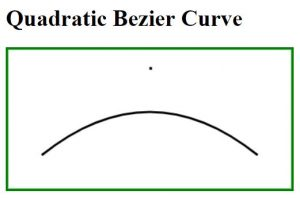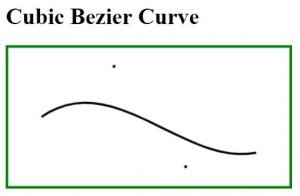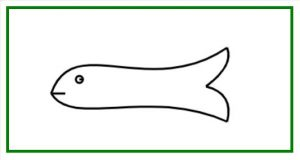# HTML | Canvas Draw Bezier Curve

• Last Updated : 27 Feb, 2019

Curves on HTML canvas can be drawn using arcs, but drawing a complex diagram using arcs is quite a tedious task. In the given circumstance, Bezier curve will be very useful in providing more flexibility in drawing curves. Bezier curves on HTML canvas are drawn using a start point, one or more control point/points and an endpoint. Example: In the case of drawing a landscape, real-world objects, irregular shapes etc.
Bezier Curves can be drawn in two ways:

• Cubic Bezier Curve

Quadratic Bezier Curve: This curve is controlled by one control point.

Syntax:

```moveTo(start_pnt_X, start_pnt_Y);
```

Example: This example create a curve using quadratic bezier curve.

 ` ``<``html``>`` ` `<``head``>``    ``<``title``>``        ``Quadratic Bezier Curve``    `````` ` `<``body``> ``    ``<``h1``>Quadratic Bezier Curve``     ` `    ``<``canvas` `id``=``"CanvasOfGeeks"` `width``=``"400"` `height``=``"200"` `            ``style``=``"border:solid 4px green"``> ``     ` `    ``<``script``> ``        ``var c = document.getElementById("CanvasOfGeeks");``        ``var context = c.getContext("2d");``         ` `        ``var start_pnt_X = 50;``        ``var start_pnt_Y = 150;``        ``var cntrl_pnt_X = 200;``        ``var cntrl_pnt_Y = 30;``        ``var end_pnt_X = 350;``        ``var end_pnt_Y = 150;``         ` `        ``/* Start new path */``        ``context.beginPath();``        ``context.lineWidth=3; ``        ``context.strokeText( ".", cntrl_pnt_X, cntrl_pnt_Y);``         ` `        ``/* Starting point of the curve */``        ``context.moveTo(start_pnt_X, start_pnt_Y); ``         ` `        ``context.quadraticCurveTo(cntrl_pnt_X, ``                    ``cntrl_pnt_Y, end_pnt_X, end_pnt_Y);``                     ` `        ``/* drawing line on the canvas */``        ``context.stroke();``    `` `` `` ` `                    `

Output:Explanation:

• Pre-requisite: HTML Canvas Basics
• First Line: Reference for canvas object is stored in variable ‘c’ using DOM concept.
Second Line: Without having drawing context of canvas nothing can be drawn on it.

```var c = document.getElementById("CanvasOfGeeks");
var context = c.getContext("2d");
```
• One can change the width of line by overriding the value of “lineWidth” attribute of context object.
`context.lineWidth=3;`
• For putting a dot over the coordinate of control point.You can see the dot in the figure shown above.
`context.strokeText( ".", cntrl_pnt_X, cntrl_pnt_Y);`
• This function is used to draw a curve from the start point mentioned in function.
`context.quadraticCurveTo(cntrl_pnt_X, cntrl_pnt_Y, end_pnt_X, end_pnt_Y);`
• This function is used to move the context.
`context.moveTo(start_pnt_X, start_pnt_Y);`

Note: Please keep the control point within the canvas boundary.

Cubic Bezier Curve: This curve is controlled by two control points.

Syntax:

```moveTo(start_pnt_X, start_pnt_Y);
contex.bezierCurveTo(cntrl_pnt_1_X, cntrl_pnt_1_Y, cntrl_pnt_2_X,
cntrl_pnt_2_Y, end_pnt_X, end_pnt_y);
```

Example: This example create a curve using cubic bezier curve.

 ` ``<``html``>``      ` `<``head``>``    ``<``title``>``        ``Cubic Bezier Curve``    `````` ` `<``body``> ``    ``<``h1``>Cubic Bezier Curve``     ` `    ``<``canvas` `id``=``"CanvasOfGeeks"` `width``=``"400"` `height``=``"200"` `            ``style``=``"border:solid 4px green"``> ``     ` `    ``<``script``> ``        ``var c = document.getElementById("CanvasOfGeeks"); ``        ``var context = c.getContext("2d");``         ` `        ``var start_pnt_X = 50;``        ``var start_pnt_Y = 100;``        ``var cntrl_pnt_1_X = 150;``        ``var cntrl_pnt_1_Y = 30;``        ``var cntrl_pnt_2_X = 250;``        ``var cntrl_pnt_2_Y = 170;``        ``var end_pnt_X     = 350;``        ``var end_pnt_Y     = 150;``         ` `        ``/* Start a new Path */``        ``context.beginPath();     ``        ``context.lineWidth=3;``         ` `        ``/* Representing first control point */``        ``context.strokeText( ".", cntrl_pnt_1_X, cntrl_pnt_1_Y); ``         ` `        ``/* Representing second control point */``        ``context.strokeText( ".", cntrl_pnt_2_X, cntrl_pnt_2_Y); ``         ` `        ``/* Starting point of the curve */``        ``context.moveTo(start_pnt_X, start_pnt_Y); ``        ``context.bezierCurveTo(cntrl_pnt_1_X, cntrl_pnt_1_Y, ``            ``cntrl_pnt_2_X, cntrl_pnt_2_Y, end_pnt_X, end_pnt_Y);``         ` `        ``/* Drawing line on the canvas  */``        ``context.stroke(); ``    `` `` `` ` `                    `

Output:Example: This example draw a fish using Bezier Curve.
Input:

 ```<``html``> `` ` `<``head``>``    ``<``title``>``        ``Drawing a fish using Bezier Curve``    `````` ` `<``body``> ``    ``<``canvas` `id``=``"CanvasOfGeeks"` `width``=``"400"` `height``=``"200"` `            ``style``=``"border:solid 4px green"``> ``     ` `    ``<``script``> ``        ``var c = document.getElementById("CanvasOfGeeks"); ``        ``var context = c.getContext("2d");``         ` `        ``/* Start a new Path */``        ``context.beginPath();``        ``context.lineWidth=3;``             ` `        ``/* Upper curve of the fish, from mouth to tail */``        ``context.moveTo(60, 120);``        ``context.bezierCurveTo(90, 30, 200, 130, 310, 55);``             ` `        ``/* Lower curve of the fish, from mouth to tail */``        ``context.moveTo(60, 120);``        ``context.bezierCurveTo(90, 170, 200, 110, 310, 160);``             ` `        ``/* Upper half of tail */``        ``context.moveTo(310, 55);``        ``context.quadraticCurveTo(320, 80, 280, 110);``             ` `        ``/* lower half of tail */``        ``context.moveTo(310, 160);``        ``context.quadraticCurveTo(320, 120, 280, 110);``             ` `        ``/* Eye of the fish */``        ``context.moveTo(100, 100);``        ``context.arc(100, 100, 5, 0, 2*Math.PI);``             ` `        ``/* Mouth of the fish */``        ``context.moveTo(60, 120);``        ``context.lineTo(80, 120);``        ``context.stroke();``    `` `` `` ` `                    `

Output:My Personal Notes arrow_drop_up